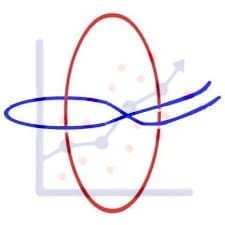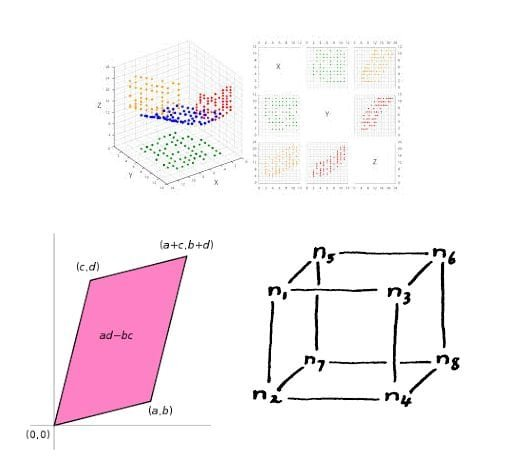Categories
College Mathematics

# Rank:IIT JAM 2018 PROBLEM 9

This problem appeared in IIT JAM 2018. This problem appeared from the brach of vector space which also requied basic knowledge from rank of a matrix.

[h5p id="15"]

# Understand the problem

Consider the vector space V over$\mathbb{R}$of the polynomial functions of degree less than or equal to 3 defined on$\mathbb{R}$. Let$T : V \longrightarrow V$defined by \$latex (Tf)(x) = f(x)-xf'(x). Then the rank of T is  (a) 1  (b) 2 (c) 3 (d) 4
##### Source of the problem
IIT JAM 2018 Problem 9
Vector Space
Easy
##### Suggested Book
Abstract Algebra By S.K Mapa

Do you really need a hint? Try it first!
Rank(T) = dim(Range(T)) There is one easy way to calculate rank of every linear transformation. Step 1:  Take by basis$\beta= \{e_1,....,e_n\}$of the vector space$V$. Step 2: Write down the matrix$[T]_{\beta}^{\beta}$Step 3: Calculate the rank of the matrix$[T]_{\beta}^{\beta}$Now can you follow these steps to get the answer?
Standard Basis of$V$is$\{1,x,x^{2},x^{3}\} = \beta$$(Tf) (x) =f(x) - xf^{'}(x)$$(T1) (x) = 1 - 0 = 1$;$(Tx) (x) = x - x = 0$;$(T x^{2}) (x)= x^{2} - 2x^{2} = -x^{2}$;$(T x^{3}) (x) = -2x^{3}$So,$[T]_{\beta}^{\beta} = \begin{pmatrix} 1 & 0 & 0 & 0 \\ 0 & 0 & 0 & 0\\ 0 & 0 & -1 & 0\\ 0 & 0 & 0 & -2 \\ \end{pmatrix}$Hence the rank is$3$

# Connected Program at Cheenta

#### College Mathematics Program

The higher mathematics program caters to advanced college and university students. It is useful for I.S.I. M.Math Entrance, GRE Math Subject Test, TIFR Ph.D. Entrance, I.I.T. JAM. The program is problem driven. We work with candidates who have a deep love for mathematics. This program is also useful for adults continuing who wish to rediscover the world of mathematics.

# Similar Problems## ISI MStat PSB 2006 Problem 8 | Bernoullian Beauty

This is a very simple and regular sample problem from ISI MStat PSB 2009 Problem 8. It It is based on testing the nature of the mean of Exponential distribution. Give it a Try it !## ISI MStat PSB 2009 Problem 8 | How big is the Mean?

This is a very simple and regular sample problem from ISI MStat PSB 2009 Problem 8. It It is based on testing the nature of the mean of Exponential distribution. Give it a Try it !## ISI MStat PSB 2009 Problem 4 | Polarized to Normal

This is a very beautiful sample problem from ISI MStat PSB 2009 Problem 4. It is based on the idea of Polar Transformations, but need a good deal of observation o realize that. Give it a Try it !## ISI MStat PSB 2009 Problem 6 | abNormal MLE of Normal

This is a very beautiful sample problem from ISI MStat PSB 2009 Problem 6. It is based on the idea of Restricted Maximum Likelihood Estimators, and Mean Squared Errors. Give it a Try it !## ISI MStat PSB 2009 Problem 3 | Gamma is not abNormal

This is a very simple but beautiful sample problem from ISI MStat PSB 2009 Problem 3. It is based on recognizing density function and then using CLT. Try it !## ISI MStat PSB 2009 Problem 1 | Nilpotent Matrices

This is a very simple sample problem from ISI MStat PSB 2009 Problem 1. It is based on basic properties of Nilpotent Matrices and Skew-symmetric Matrices. Try it !## ISI MStat PSB 2006 Problem 2 | Cauchy & Schwarz come to rescue

This is a very subtle sample problem from ISI MStat PSB 2006 Problem 2. After seeing this problem, one may think of using Lagrange Multipliers, but one can just find easier and beautiful way, if one is really keen to find one. Can you!

## Problem on Inequality | ISI – MSQMS – B, 2018 | Problem 2a

Try this problem from ISI MSQMS 2018 which involves the concept of Inequality. You can use the sequential hints provided to solve the problem.## Data, Determinant and Simplex

This problem is a beautiful problem connecting linear algebra, geometry and data. Go ahead and dwelve into the glorious connection.

## Problem on Integral Inequality | ISI – MSQMS – B, 2015

Try this problem from ISI MSQMS 2015 which involves the concept of Integral Inequality and real analysis. You can use the sequential hints provided to solve the problem.

This site uses Akismet to reduce spam. Learn how your comment data is processed.